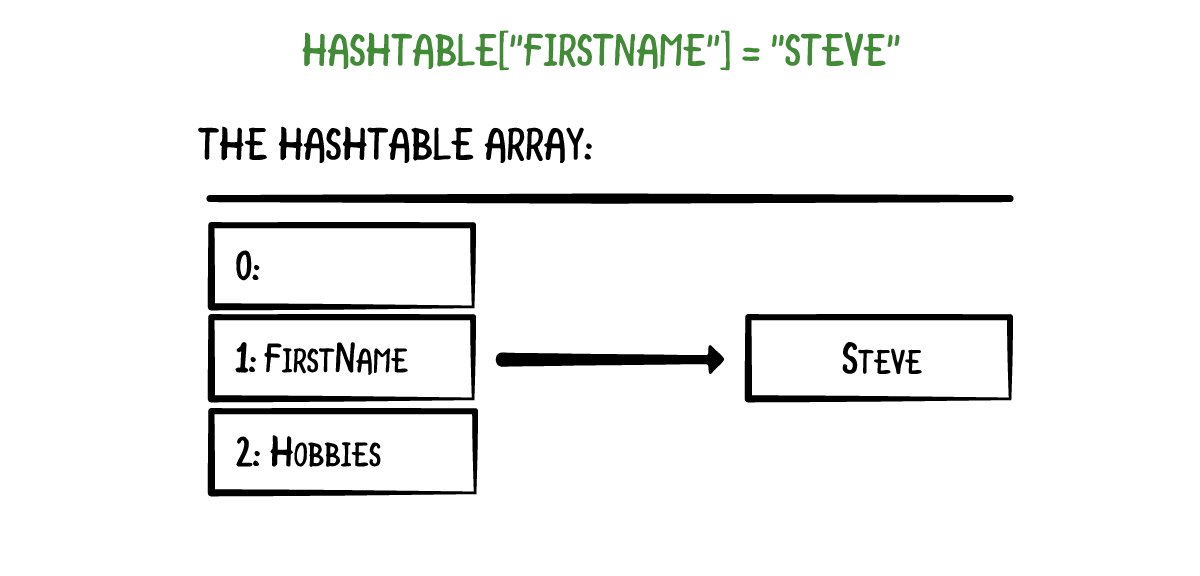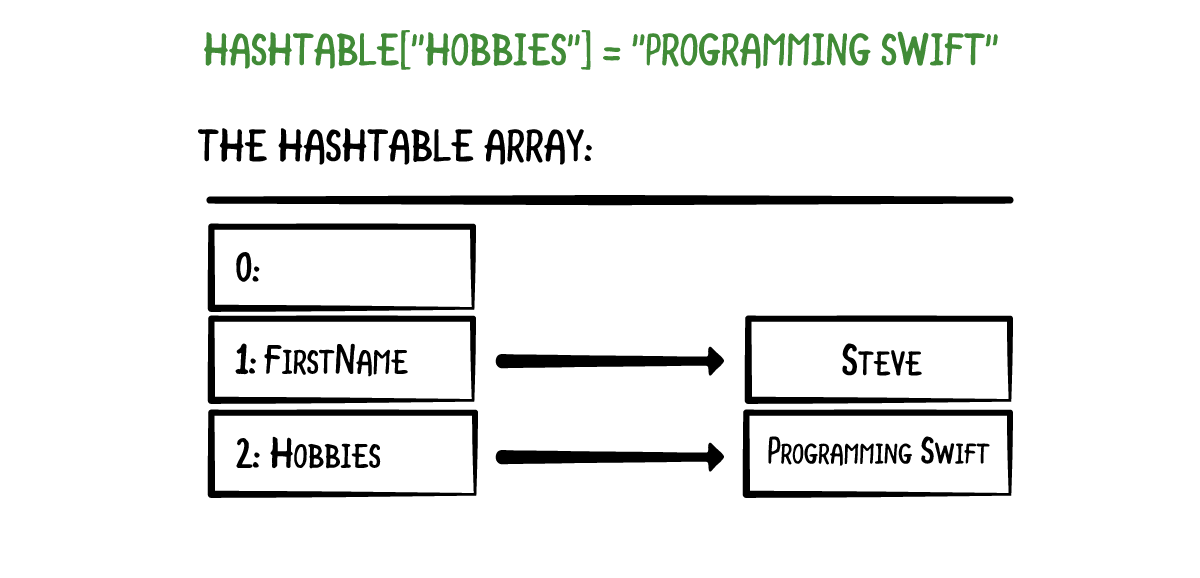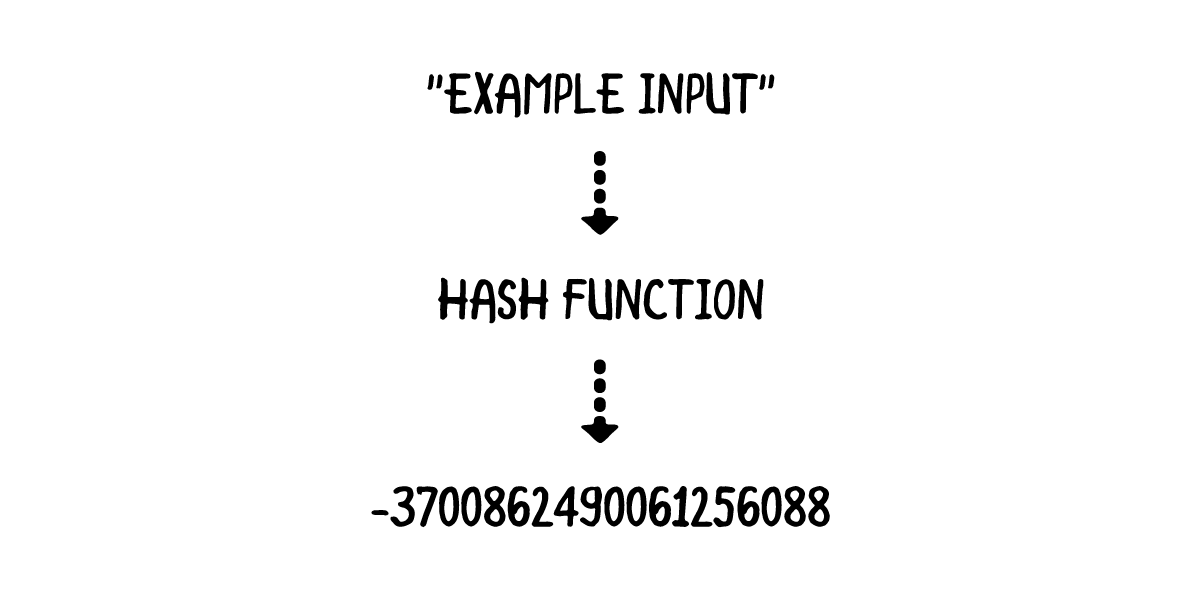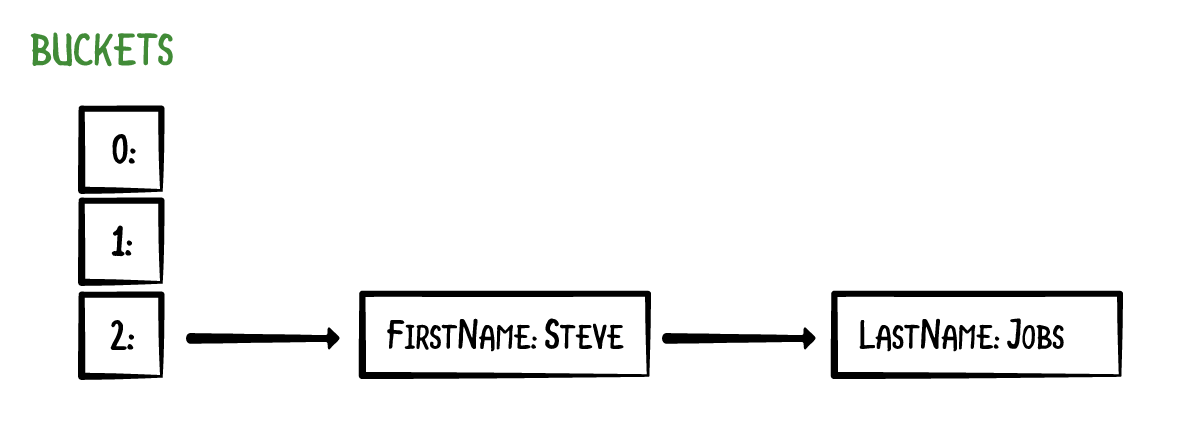# Swift Algorithm Club: Hash Tables

Learn how to implement a hash table data structure in Swift, in this step-by-step tutorial from the Swift Algorithm Club. By Kelvin Lau.

Leave a rating/review
Save for later
Share

## Swift Algorithm Club: Hash Tables

20 mins

The Swift Algorithm Club is an open source project to implement popular algorithms and data structures in Swift.

Every month, the SAC team will feature a cool data structure or algorithm from the club in a tutorial on this site. If your want to learn more about algorithms and data structures, follow along with us!

In this tutorial, you’ll learn how to implement a Swift hash table. This data structure was first implemented by Matthijs Hollemans, and is now refactored for tutorial format.

## Getting Started

As a Swift developer, you’re familiar with the `Dictionary` structure. If you’re also a Kotlin developer, you would be familiar with the `HashMap` class. The underlying data structure that powers both of these constructs is the hash table data structure.

The Swift dictionary is a collection of key-value pairs. Storing an value in a dictionary requires you to pass in a value with a key:

```var dictionary: [String: String] = [:]

dictionary["firstName"] = "Steve"

// retrieve value for a key
dictionary["firstName"] // outputs "Steve"
```

Underneath the hood, the dictionary will pass the key-value pair to a hash table for storage. In this tutorial, you’ll learn about a basic implementation of the hash table and learn about its performance.

## Hash Tables

A hash table is nothing more than an array. Initially, this array is empty. When you put a value into the hash table under a certain key, it uses that key to calculate an index in the array. Here is an example:In this example, the key `"firstName"` maps to array index 1.

Adding a value under a different key puts it at another array index:The trick is how the hash table calculates those array indices. That is where the hashing comes in. When you write the following statement,

```hashTable["firstName"] = "Steve"
```

the hash table takes the key “firstName” and asks it for its `hashValue` property. Hence, keys must conform to the `Hashable` protocol.

### Hash Functions

When you write `"firstName".hashValue`, it returns a big integer: `-8378883973431208045`. Likewise, `"hobbies".hashValue` has the hash value `477845223140190530`.

Hash values are calculated by a hash function, which takes some input and returns an integer:To achieve a better understanding of how hash functions work, you’ll implement two simple hash functions of your own. Open up a playground and write the following in the playground page:

```func naiveHash(_ string: String) -> Int {
let unicodeScalars = string.unicodeScalars.map { Int(\$0.value) }
return unicodeScalars.reduce(0, +)
}
```

This above hash function uses the unicode scalar representation of each character and sums it up. Write the following into the playground page:

```naiveHash("abc") // outputs 294
```

While this hash function does map a string to an integer value, it’s not very good. See why by writing the following:

```naiveHash("bca") // also outputs 294
```

Since `naiveHash` only sums up the unicode scalar representation of each character, any permutation of a specific string will yield the same result.

A single lock is being opened by many keys! This isn’t good. A significantly improved hash function can be obtained with just a bit more effort. Write the following in the playground page:

```// sourced from https://gist.github.com/kharrison/2355182ac03b481921073c5cf6d77a73#file-country-swift-L31
func djb2Hash(_ string: String) -> Int {
let unicodeScalars = string.unicodeScalars.map { \$0.value }
return unicodeScalars.reduce(5381) {
(\$0 << 5) &+ \$0 &+ Int(\$1)
}
}

djb2Hash("abc") // outputs 193485963
djb2Hash("bca") // outputs 193487083
```

This time, the two permutations have different hash values. The hash function implementation in the Swift Standard Library for `String` is far more complicated, and uses the `SipHash` algorithm. That's beyond the scope of this tutorial.

• If you're interested in how the strings compute the hash value, you can check out the source code here.
• Swift uses the SipHash hash function to handle many of the hash value calculations. The API to this implementation is private, but a public implementation has been done here.
Note:

### Keeping Arrays Small

`"firstName"` has a hash value of `-8378883973431208045`. Not only is this number large, it's also negative. So how do you fit it into an array?

A common way to make these big numbers suitable is to first make the hash positive and then take the modulo with the array size.

In the example earlier, the array had a size of three. The index for the `"firstName"` key becomes `abs(-8378883973431208045) % 3 = 1`. You can calculate the array index for `"hobbies"` is 2.

Using hashes in this manner is what makes the dictionary efficient; To find an element in the hash table, you must hash the key to get an array index and then look up the element in the underlying array. All these operations take a constant amount of time, so inserting, retrieving, and removing are all `O(1)`.

Note: It is difficult to predict where in the array your objects end up. Hence, dictionaries do not guarantee any particular order of the elements in the hash table.

### Avoiding Collisions

There is one problem. Taking the modulo of the hash value with a number can lead to a same value. Here's one such example:

```djb2Hash("firstName") % 2 // outputs 1
djb2Hash("lastName") % 2 // outputs 1
```

This example is a little contrived, but serves the purpose of highlighting the possibility of a hash mapping to the same index. This is known as a collision. A common way to handle collisions is to use chaining. The array looks as follows:With chaining, keys and their values are not stored directly in the array. Instead, each array element is a list of zero or more key-value pairs. The array elements are usually called buckets, and the lists are called the chains. Here we have 3 buckets, and the bucket at index 2 has a chain. The other buckets are empty.

### Retrieving Items

Here's an example of retrieving an item from the hash table:

```let x = hashTable["lastName"]
```

The hash table first hashes the key `"lastName"` to calculate the array index, which is 2. Since bucket 2 has a chain, you step through the list to find the value with the key `"lastName"`. This is done by comparing the keys using a string comparison. The hash table checks that the key belongs to the last item in the chain and returns the corresponding value, `"Jobs"`.

Common ways to implement this chaining mechanism are to use a linked list or another array. Chains should not become long because looking up items in the hash table would become a slow process. Ideally, you would have no chains at all, but in practice it is impossible to avoid collisions. You can improve the odds by giving the hash table enough buckets and usin high-quality hash functions.

Note: An alternative to chaining is "open addressing". The idea is this: if an array index is already taken, we put the element in the next unused bucket. This approach has its own upsides and downsides.Kelvin Lau

Author